# II Molar Conversions A Molar Mass Find the

• Slides: 12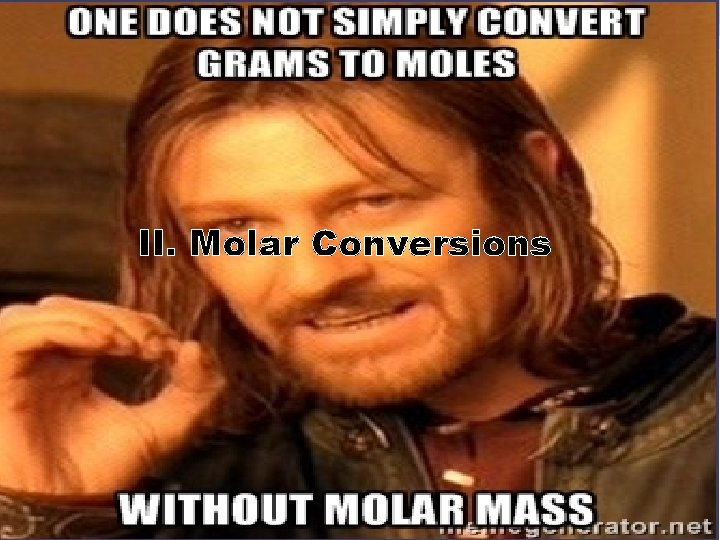II. Molar ConversionsA. Molar Mass • Find the mass of one mole of the following atoms/compounds (molar mass) 1 mole of H 2 O = 1 mole of CO 2 = 1 mole of H 3 PO 4 =B. Grams Moles How many moles is 350 g of CO 1 mole CO =_____gB. Grams Moles What is the mass of 6. 8 moles of Mg. Cl 2 1 mole Mg. Cl 2=_____gC. Grams Moles Particles How many particles are there in 24 g of Fe. F 3? (hint: write two unit conversions before solving) 1 mole of Fe. F 3 = 1 mole =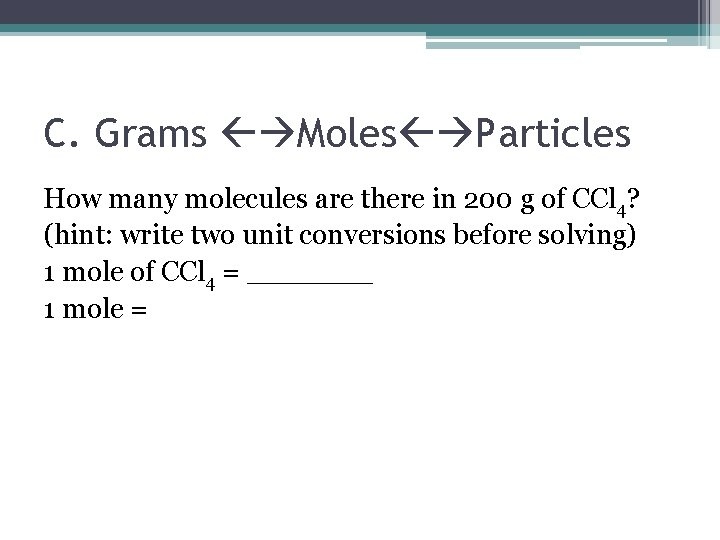C. Grams Moles Particles How many molecules are there in 200 g of CCl 4? (hint: write two unit conversions before solving) 1 mole of CCl 4 = _______ 1 mole =C. Grams Moles Particles How many grams of H 2 are in 9. 4 x 1025 molecules of H 2? (hint: write two unit conversions before solving) 1 mole of H 2 = 1 mole =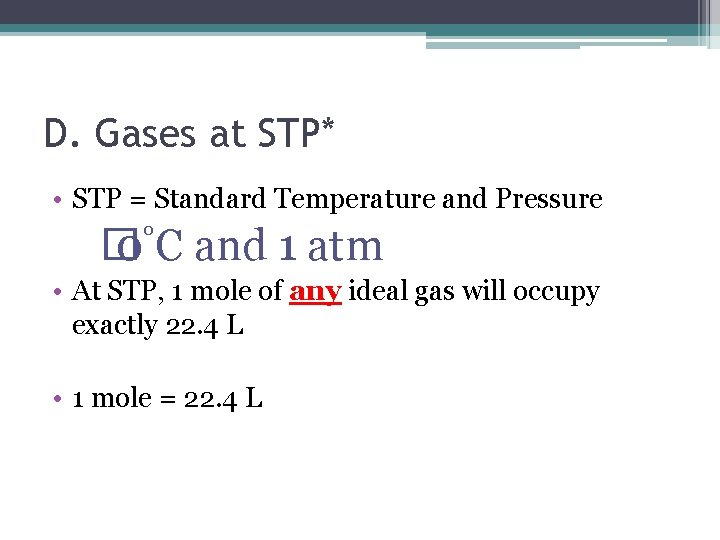D. Gases at STP* • STP = Standard Temperature and Pressure � 0°C and 1 atm • At STP, 1 mole of any ideal gas will occupy exactly 22. 4 L • 1 mole = 22. 4 L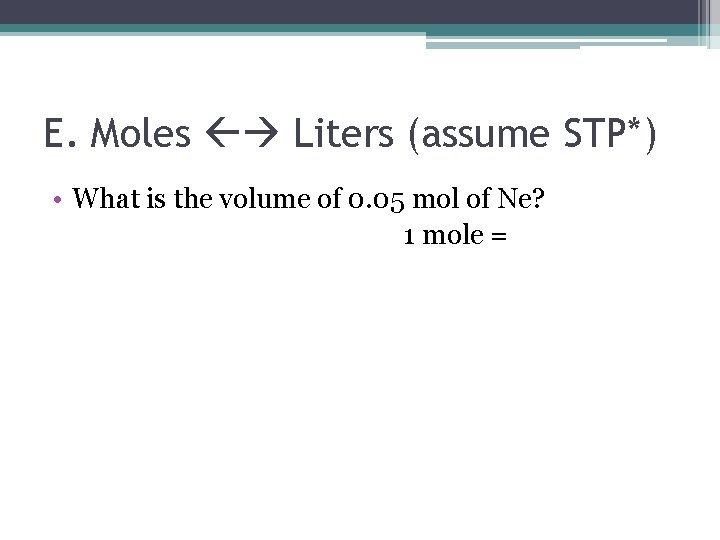E. Moles Liters (assume STP*) • What is the volume of 0. 05 mol of Ne? 1 mole =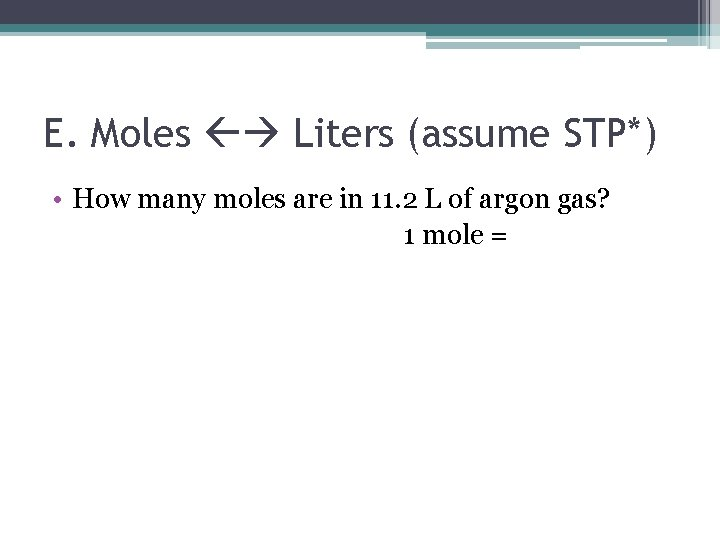E. Moles Liters (assume STP*) • How many moles are in 11. 2 L of argon gas? 1 mole =F. Find the Molar Mass • A 4. 8 -mole sample of an unknown compound has a mass of 192 g. Find the molar mass of the compound. Units for molar mass = ___ /___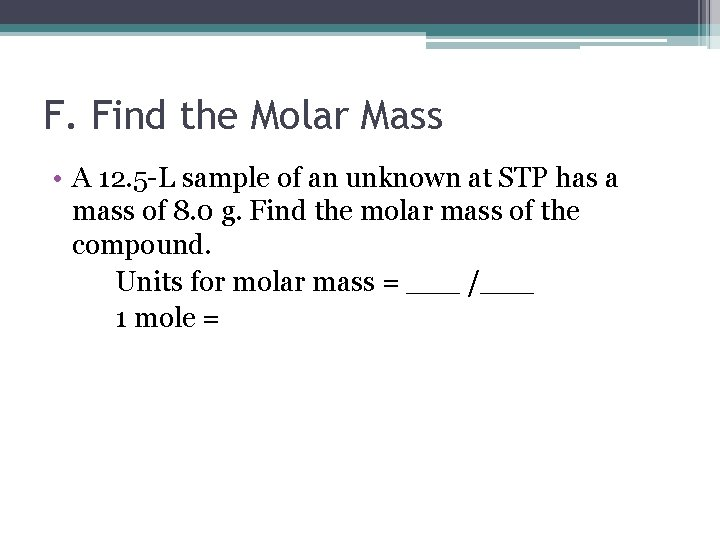F. Find the Molar Mass • A 12. 5 -L sample of an unknown at STP has a mass of 8. 0 g. Find the molar mass of the compound. Units for molar mass = ___ /___ 1 mole =